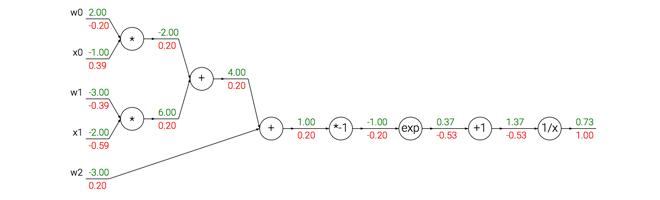## 原文如下：

• 简介
• 简单表达式和理解梯度
• 复合表达式，链式法则，反向传播
• 直观理解反向传播
• 模块：Sigmoid例子
• 反向传播实践：分段计算
• 回传流中的模式
• 用户向量化操作的梯度
• 小结

## 简单表达式和理解梯度## 使用链式法则计算复合表达式

# 设置输入值
x = -2; y = 5; z = -4

# 进行前向传播
q = x + y # q becomes 3
f = q * z # f becomes -12

# 进行反向传播:
# 首先回传到 f = q * z
dfdz = q # df/dz = q, 所以关于z的梯度是3
dfdq = z # df/dq = z, 所以关于q的梯度是-4
# 现在回传到q = x + y
dfdx = 1.0 * dfdq # dq/dx = 1. 这里的乘法是因为链式法则
dfdy = 1.0 * dfdq # dq/dy = 1


————————————————————————————————————————

————————————————————————————————————————

## 模块化：Sigmoid例子———————————————————————————————————————

————————————————————————————————————————w = [2,-3,-3] # 假设一些随机数据和权重
x = [-1, -2]

# 前向传播
dot = w*x + w*x + w
f = 1.0 / (1 + math.exp(-dot)) # sigmoid函数

# 对神经元反向传播
ddot = (1 - f) * f # 点积变量的梯度, 使用sigmoid函数求导
dx = [w * ddot, w * ddot] # 回传到x
dw = [x * ddot, x * ddot, 1.0 * ddot] # 回传到w
# 完成！得到输入的梯度


## 反向传播实践：分段计算x = 3 # 例子数值
y = -4

# 前向传播
sigy = 1.0 / (1 + math.exp(-y)) # 分子中的sigmoi          #(1)
num = x + sigy # 分子                                    #(2)
sigx = 1.0 / (1 + math.exp(-x)) # 分母中的sigmoid         #(3)
xpy = x + y                                              #(4)
xpysqr = xpy**2                                          #(5)
den = sigx + xpysqr # 分母                                #(6)
invden = 1.0 / den                                       #(7)
f = num * invden # 搞定！                                 #(8)


┗|｀O′|┛ 嗷~~，到了表达式的最后，就完成了前向传播。注意在构建代码s时创建了多个中间变量，每个都是比较简单的表达式，它们计算局部梯度的方法是已知的。这样计算反向传播就简单了：我们对前向传播时产生每个变量(sigy, num, sigx, xpy, xpysqr, den, invden)进行回传。我们会有同样数量的变量，但是都以d开头，用来存储对应变量的梯度。注意在反向传播的每一小块中都将包含了表达式的局部梯度，然后根据使用链式法则乘以上游梯度。对于每行代码，我们将指明其对应的是前向传播的哪部分。

# 回传 f = num * invden
dnum = invden # 分子的梯度                                         #(8)
dinvden = num                                                     #(8)
# 回传 invden = 1.0 / den
dden = (-1.0 / (den**2)) * dinvden                                #(7)
# 回传 den = sigx + xpysqr
dsigx = (1) * dden                                                #(6)
dxpysqr = (1) * dden                                              #(6)
# 回传 xpysqr = xpy**2
dxpy = (2 * xpy) * dxpysqr                                        #(5)
# 回传 xpy = x + y
dx = (1) * dxpy                                                   #(4)
dy = (1) * dxpy                                                   #(4)
# 回传 sigx = 1.0 / (1 + math.exp(-x))
dx += ((1 - sigx) * sigx) * dsigx # Notice += !! See notes below  #(3)
# 回传 num = x + sigy
dx += (1) * dnum                                                  #(2)
dsigy = (1) * dnum                                                #(2)
# 回传 sigy = 1.0 / (1 + math.exp(-y))
dy += ((1 - sigy) * sigy) * dsigy                                 #(1)
# 完成! 嗷~~


## 回传流中的模式

——————————————————————————————————————————

——————————————————————————————————————————

## 用向量化操作计算梯度

# 前向传播
W = np.random.randn(5, 10)
X = np.random.randn(10, 3)
D = W.dot(X)

# 假设我们得到了D的梯度
dD = np.random.randn(*D.shape) # 和D一样的尺寸
dW = dD.dot(X.T) #.T就是对矩阵进行转置
dX = W.T.dot(dD)


## 小结

• 对梯度的含义有了直观理解，知道了梯度是如何在网络中反向传播的，知道了它们是如何与网络的不同部分通信并控制其升高或者降低，并使得最终输出值更高的。

• 讨论了分段计算在反向传播的实现中的重要性。应该将函数分成不同的模块，这样计算局部梯度相对容易，然后基于链式法则将其“链”起来。重要的是，不需要把这些表达式写在纸上然后演算它的完整求导公式，因为实际上并不需要关于输入变量的梯度的数学公式。只需要将表达式分成不同的可以求导的模块（模块可以是矩阵向量的乘法操作，或者取最大值操作，或者加法操作等），然后在反向传播中一步一步地计算梯度。

## 译者反馈

1. 转载须全文转载注明原文链接，否则保留维权权利；
2. 知乎上关于反向传播的问题很多，希望知友们能点赞帮助本文广泛传播为更多人解惑
3. 请知友们通过评论和私信等方式批评指正，贡献者均会补充提及；
4. 感谢知友roach sinai郑申海陈一的细节修改建议。